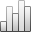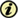Acta Stereologica

0351-580XMore Statisticssince 05 December 2013 :
View(s): 338 (6 ULiège)
Edmund M. Glaser & P. David Wilson

### A comparison of three estimators of the coefficient of error of optical fractionator cell count estimates

(Volume 18 (1999) — Number 1 - May 1999)
Article#### Abstract

The optical fractionator is a design-based two-stage systematic sampling method that is used to estimate the number of cells in populations that are too large to count exhaustively. It counts the cells found in optical disectors that have been systematically sampled in serial sections. Computer simulation was used to investigate three methods for estimating the coefficient of error (CE), the precision of a population size estimate, obtained from a single optical fractionator sample. The methods were: the original estimation equation of Gundersen and Jensen (1987), its nugget effect modification (West et al, 1996), and the method of Scheaffer, Mendenhall, and Ott (1996), which has not been used in stereology. It is difficult to evaluate the estimated precision of population cell count estimates by using biological tissue samples. They do not permit a comparison of an estimated CE with the true CE. Computer simulation does permit such comparisons while avoiding the observational biases inherent in working with biological tissue. The estimated CE's were evaluated in tests of three types of non-random cell population distribution and one random cell population distribution. The non-random population distributions varied according to both section and disector location within the section. Two were sinusoidal and one was linearly increasing; in all three there was a 6-fold difference between the high and low intensities, i.e., expected cell counts per disector. The sinusoidal distributions produced either a peak or a depression of cell intensity at the center of the simulated region. The linear cell intensity gradually increased from the beginning to the end of the region that contained the cells. The random population distribution had constant cell intensity over the region. A test condition was defined by its population distribution, the period between consecutive sampled sections and the spacing between consecutive sampled disectors. There were 1,000 trials of each test condition. In each trial were calculated the true CE of the expected cell count estimate and the three CE estimates obtained by applying the SMO and both GJ equations to single two-stage systematic samples. The estimated CE's were compared with the true CE's for all the population distributions. The CE estimates obtained by the SMO estimator were found to be closer to the true CE's and had less scatter than those of the nugget-modified GJ estimator. Both had small positive bias and comparable scatter. The CE estimates obtained by the unmodified GJ estimator exhibited large negative bias and large scatter. In all the population distributions tested, the average true CE was very nearly proportional to 1/√Q̅t , where Q̅t is the average number of sampled cells.

Keywords : cell count estimate precision, computer simulation, nugget effect, optical disector, optical fractionator, systematic sampling